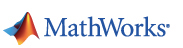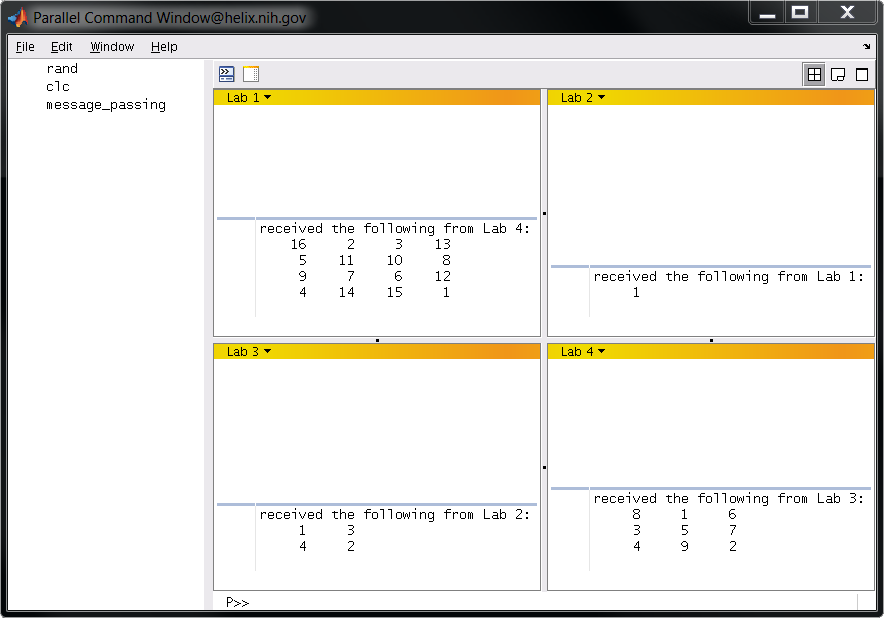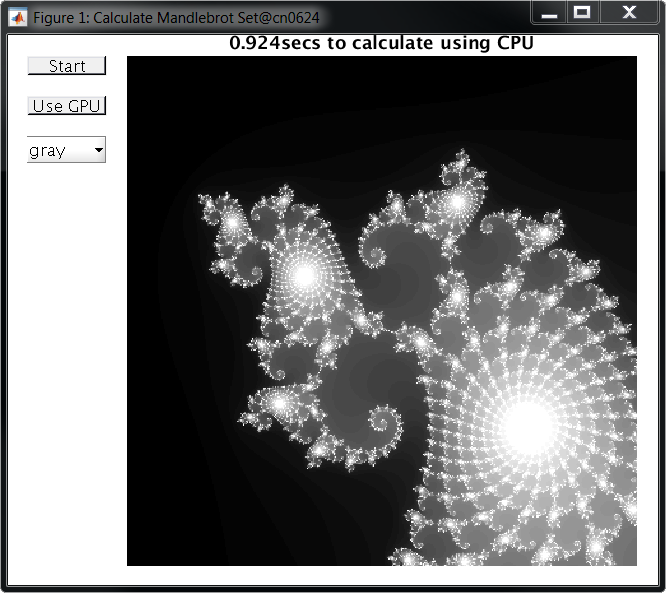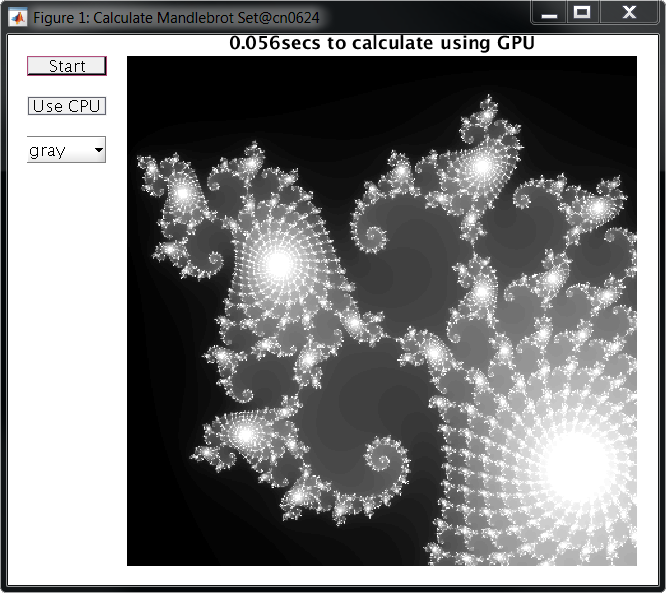MATLAB Parallel Computing Toolbox
 Quick Links DescriptionThe MATLAB Parallel Computing Toolbox enables you to develop distributed and parallel MATLAB applications and execute them on multiple workers. Workers are multiple instances of MATLAB that run on individual cores. Please note the following: In it's present configuration, the Parallel Computing Toolbox does not scale beyond a single node. This will allow your job to run on up to 28 cores on the norm partition, which will be sufficient for many jobs. But to run distributed jobs on multiple nodes, use sbatch or swarm. Within MATLAB and in online documentation this toolbox is referred to as the Parallel Computing Toolbox. Within the licensing software it is referred to as the Distributed Processing Toolbox. There is a related MathWorks product that is not currently installed on our systems that extends the functionality of the Parallel Computing Toolbox called the Distributed Computing Server. This nomenclature sometimes causes confusion. IMPORTANT: (November 2021) Biowulf users now have access to unlimited Matlab licenses and all toolboxes The NIH HPC Staff is pleased to announce a new Matlab license model that provides the following advantages to Biowulf Matlab users: (1) access to all Matlab toolboxes, (2) unlimited number of Matlab licenses, (3) the ability to run batch jobs without using the Matlab compiler, and (4) the ability to submit large numbers of Matlab batch jobs. As before, interactive Matlab jobs are still possible, and are limited to two sinteractive sesssions.

Web sites

The examples on this page assume you are running the MATLAB IDE in an X Windows session. To run them, start an interactive MATLAB session on a Biowulf compute node and allocate multiple CPUs (User input in bold.)

```[user@biowulf ~]\$ sinteractive --cpus-per-task=56 --mem=245g #this will take a whole node on the norm partition
salloc.exe: Pending job allocation 17637311
salloc.exe: job 17637311 queued and waiting for resources
salloc.exe: job 17637311 has been allocated resources
salloc.exe: Granted job allocation 17637311
salloc.exe: Waiting for resource configuration
salloc.exe: Nodes cn0055 are ready for job
srun: error: x11: no local DISPLAY defined, skipping

[user@cn0055 ~]\$ matlab&
```

If your X Windows client is working properly you should now see the MATLAB IDE.

Running a process in in the background

The simplest type of distributed computing involves running a process in the background while you continue to work in your interactive session uninterrupted.

For this and some following examples, I have a created a MATLAB function that will make a short movie of drifting sin wave gratings. (These "Gabor patches" are important stimuli in the field of visual neuroscience.) The function picks some random parameters and generates a .avi file. It takes anywhere from ~10 to 60 seconds to run. You can see the source code here. If you want to run these examples yourself, just copy the text into a file called gabor_patch_avi.m

You can run gabor_patch_avi in the background like so:

```>> jid = batch('gabor_patch_avi');
```

After a moment, your command prompt should return allowing you to continue working in your interactive session. After another moment you should see a randomly named .avi file appear in you current directory and begin to grow indicating that gabor_patch_avi is running in the background.

Make sure you have allocated enough CPUs and memory to support your processes running in the background. As a general rule, each MATLAB process should have it's own core (2 cpus). For more info on running MATLAB code using batch (including running batch jobs that generate input and output) see the MathWorks documentation.

Distributed (non-communicating) computations

One way to start a distributed set of processes is to initiate cluster and job objects and pass them function handles (although for a much simpler solution see parfor loops below.) The script below will run 4 simultaneous instances of gabor_patch_avi on MATLAB workers in the background, leaving you free to keep working without interruption.

```% set this to the number you want
job_num = 4;

% make the cluster object
clust_obj = parcluster;
clust_obj.NumWorkers = job_num;

% make the job object
job_obj = clust_obj.createJob;
for ii = 1:job_num
end

% submit the jobs
job_obj.submit;
```

The 0 input to createTask indicates that gabor_patch_avi produces zero outputs. If your function takes input, you can add a cell array to your createTask call with one cell for every input like so:

```job_obj.createTask(@my_function, 1, {input1, input2})
```

In this case, I have also indicated that my_function produces one output. If your job creates output, you can retrieve it with fetchOutputs like so:

```my_output = fetchOutputs(job_obj);
```

The outputs will appear in a cell array with each cell containing the output from one job. Check the MathWorks documentation for more info on using parcluster and createTask.

As a general rule, you should allocate a single core (2 cpus) for each MATLAB worker. For instance, to run this example, make sure you have allocated at least 8 CPUs in your sinteractive command.

Parallel (communicating) computations

MATLAB processes running on multiple cores can share memory and pass messages to one another. The simplest way to initiate a parallel computation in MATLAB is to use a parfor loop.

parfor (parallel for) loops

The preceding example of a distributed job could be simplified by starting a parallel pool of MATLAB workers and then writing a simple parfor loop. (The only drawback with this approach is that you must wait for the code to finish executing before you can start using MATLAB again.) We could do this at the MATLAB command prompt like so:

```>> my_pool = parpool(4);
Starting parallel pool (parpool) using the 'local' profile ... connected to 4 workers.
>> parfor ii = 1:4, gabor_patch_avi, end
>> delete(my_pool)
Parallel pool using the 'local' profile is shutting down.
```

But parfor loops are even more powerful than this because the workers can share memory.

To illustrate this, let's load a directory full of images into one variable in the MATLAB workspace. We'll then use the imsharp function from the Image Processing toolbox to sharpen all of the images. First, we will do this in a for loop, and then we'll repeat this process in a parfor loop.

The following code will find all images in a given directory with a .png extension and will load them into a 4 dimensional matrix (height in pixels X width in pixels X RGB values X image number). It assumes all images are the same size. Copy the code into a file called make_image_stack.m.

```function image_stack = make_image_stack(directory)

% find all files in the directory with the .png extension
pic_list = dir(fullfile(directory, '*.png'));
imN      = length(pic_list);

% load one picture to get the size and preallocate
[xx, yy, zz] = size(test_pic);
image_stack  = zeros([xx, yy, zz, imN], 'uint8');

% load all the pictures in a loop
for ii = 1:length(pic_list)
end
```

Once you've saved this code to a .m file in your working directory, you can use it to load images from the /data/classes directory like so:

```>> directory = '/data/classes/matlab/swarm_example/lots-o-images/';
>> image_stack = make_image_stack(directory);
```

You should now have a variable in your workspace called image_stack. It contains the frames of a short movie. You can view the movie by entering the following:

```>> implay(image_stack)
```
As you can see, it's a bit blurry. Let's use imsharp in a loop to sharpen all of the frames. First, copy the following code to a file called sharpen_image_stack.m.
```function stack_out = sharpen_image_stack(stack_in, sharpen_level)

% how many images do we have?
[~,~,~, imN] = size(stack_in);

% loop through the images and sharpen each one
for ii = 1:imN
stack_in(:,:,:, ii) = imsharpen(stack_in(:,:,:, ii),...
'Amount', sharpen_level);
end

stack_out = stack_in;
```

Right now, the code does not run in parallel. You can run it and time its execution like so:

```>> tic, sharp_stack = sharpen_image_stack(image_stack, 5); toc
Elapsed time is 39.941003 seconds.
```

It should take between 30 and 40 seconds to run. You can view the result with implay(sharp_stack). Instead of sharpening each frame of this movie sequentially, you can turn it into a parallel job so that multiple MATLAB workers sharpen different frames of the movie simultaneously. To do so, first start a pool of MATLAB workers with the parpool command:

```>> parpool

ans =

Pool with properties:

Connected: true
NumWorkers: 16
Cluster: local
AttachedFiles: {}
IdleTimeout: 30 minute(s) (30 minutes remaining)
SpmdEnabled: true
```

By default parpool starts 16 workers. You can specify fewer workers with parpool(N) where N is the number of workers.

Now change the for loop in sharpen_image_stack to a parfor loop like so. (Change in italics):

```% load all the pictures in a loop
parfor ii = 1:length(pic_list)
end
```

Your code will now execute more quickly.

```>> tic, sharp_stack = sharpen_image_stack(image_stack, 5); toc
Elapsed time is 9.060358 seconds.
```

In this example, MATLAB workers are reading from the same variable in memory and writing to another shared memory location. You could also change the for loop in make_image_stack to a parfor loop for a small speed boost when first constructing the image_stack. In that example, the workers would also be sharing memory by writing to the same variable in the MATLAB workspace.

A few notes on parfor loops:

• In this example our computation was only ~4x faster with the parfor loop, even though we initiated 16 MATLAB workers. MATLAB takes advantage of built-in code optimizations when performing imsharp in a for loop. It's unable to use these optimizations when running multiple instances of imsharp in the parfor loop. Because of this tradeoff, it actually takes slightly longer to run sharpen_image_stack on 2 workers than when just using a plain for loop. Some MATLAB jobs (like running gabor_patch_avi above) may approach a 16x performance boost with 16 workers while others (like sharpen_image_stack) might not scale as well.

• There is overhead associated with initiating a pool of MATLAB workers. But that overhead need not be incurred every time a parfor loop is executed. We could run sharpen_image_stack as many times as we wanted after a single pool of workers was initiated. One could also include a line like try, parpool; end at the top of a parallel function. This statement would only be executed in the event that a pool of MATLAB workers was not already initiated.

• MATLAB error checks the body of a parfor loop and refuses to execute the code if there is any possibility that the workers will interfere with one another. One good rule of thumb is that if you can encapsulate the body of a parfor loop in a function the workers will not interfere with one another. In fact, behind the scenes MATLAB actually attempts to package the body of your parfor loop into a function and, failing this, refuses to run your code.

• spmd (single program multiple data)

A parfor loop is a special instance of spmd. If you wanted to rewrite the parfor example above using spmd it might look something like this.

```function stack_out = spmd_sharpen_image_stack(stack_in, sharpen_level)

% preallocate for later
stack_out = stack_in;

% how many images do we have?
[xx, yy, zz, imN] = size(stack_in);

% contents of spmd are executed on each worker
spmd

% with spmd we must exert low-level control over each worker including
% manually determining the array indices that each worker has access to

zero_i = labindex - 1; % zero based index is easier for some things

% which images should each worker analyze?
chunkN = imN ./ numlabs;
start  = round(chunkN * zero_i) + 1;
fin    = round(chunkN *(zero_i + 1));

if labindex < numlabs                   % if it's not the last worker...
chunk = stack_in(:,:,:, start:fin); % take the correct images to analyze
else                                    % but if it is the last worker...
chunk = stack_in(:,:,:, start:end); % just take the leftovers
end

[~,~,~, subimN] = size(chunk);
for ii = 1:subimN
chunk(:,:,:, ii) =...
imsharpen(chunk(:,:,:, ii),'Amount', sharpen_level);
end

end

clear stack_in % save memory

% the output data type (chunk) is a Composite.  it's an object that can be
% indexed similar to a cell array and it contains the output from each
% worker at the given index.  we have to reconstruct it back into a Double
stack_out = zeros([xx, yy, zz, imN], 'uint8');
ct = 1;
for ii = 1:length(chunk)

this_chunk = chunk{ii}; % Composite objects only support simple subscripting :-(
[~,~,~, subimN] = size(this_chunk);
for jj = 1:subimN
stack_out(:,:,:, ct) = this_chunk(:,:,:, jj);
ct = ct+1;
end

end
```

The parfor example is clearly superior in this case being easier to read, write, and debug. This spmd example also takes slightly longer to execute because the resultant Composite data type must be converted back to a Double matrix.

But spmd also grants increased flexibility. Within an spmd block the variable numlabs provides the number of workers in the current parallel pool, and the variable labindex provides the index of the current worker. This allows you to make the same block of code operate on different data. spmd also allows for explicit message passing between workers. Consider this code which passes messages between workers in a "round robin":

```function message_passing

spmd

% create a message (magic square) unique to each worker
my_message = magic(labindex);

% pass messages in a round robin
right_neighbor = mod(labindex, numlabs)   + 1; % mablab is base 1 indexed
left_neighbor  = mod(labindex-2, numlabs) + 1;
labSend(my_message, right_neighbor);

% print the message that was just received
fprintf('received the following from Lab %i:\n', left_neighbor)
disp(neighbors_message)

end
```

The functions labSend and labRecieve allow messages (data) to be passed between workers. When run using 4 workers, this code produces the following output:

```>> parpool(4);
Starting parallel pool (parpool) using the 'local' profile ... connected to 4 workers.
>> message_passing
Lab 1:
received the following from Lab 4:
16     2     3    13
5    11    10     8
9     7     6    12
4    14    15     1

Lab 2:
received the following from Lab 1:
1

Lab 3:
received the following from Lab 2:
1     3
4     2

Lab 4:
received the following from Lab 3:
8     1     6
3     5     7
4     9     2
```

Message passing can be very powerful, allowing you to do things like distribute large matrices that do not fit into memory on a single node across multiple nodes and carry out operations on portions of them. However, our current MATLAB license agreement only permits the Parallel Computing toolbox to be used on a single node. In practice there are very few situations in which spmd would be preferred over parfor when performing parallel computations on a single node.

pmode (interactive spmd jobs)

pmode allows you to run an entire interactive session using the spmd (single program multiple data) model. (See spmd above.) This could be useful for developing and/or debugging spmd blocks of code. To get started, issue the pmode command. This initiates a pool of parallel workers in addition to starting the GUI, so there is no need to use parpool. In this example, we use 4 workers:

```>> pmode start 4
```

You should see a GUI like the one below. Commands are entered at the location of the red arrow. In this example we are calling the function rand. Note that a different random number is generated on each worker.Let's run the preceding example (message_passing.m) in pmode. First, comment out spmd and end like so:

```function message_passing

% spmd

% create a message (magic square) unique to each worker
my_message = magic(labindex);

% pass messages in a round robin
right_neighbor = mod(labindex, numlabs)   + 1; % mablab is base 1 indexed
left_neighbor  = mod(labindex-2, numlabs) + 1;
labSend(my_message, right_neighbor);

% print the message that was just received
fprintf('received the following from Lab %i:\n', left_neighbor)
disp(neighbors_message)

% end
```

Now execute message_passing at the pmode command prompt. You should see output like the following:Note that pmode implicitly runs every command you enter as though it were in a spmd block. This is why spmd and the matching end needed to be commented out of message_passing.m. It makes no sense to call spmd within a spmd block.

By the same token, it doesn't make any sense to use parfor loops in pmode. They will not cause an error, but each parfor loop will be executed on a single worker effectively reducing them to plain old for loops. Going back to our earlier example, if you wanted to make 4 gabor patch movies in pmode with 4 workers, just enter gabor_patch_avi at the pmode prompt. The funciton will run on all 4 workers creating 4 .avi files.

GPU computing

Many computations can benefit from using Graphics Processing Units (GPUs) instead of CPUs. GPU hardware is specialized to perform extremely fast matrix computations. MATLAB (MATrix LABoratory) is software designed for efficient matrix computations, so it's only natural to use MATLAB with GPUs.

The simplest way to speed up computations using GPUs in MATLAB is to load your data onto a GPU and then use one of the many builtin functions that support gpuArray input arguments .

The following code will calculate the Mandelbrot set within a given range using either a CPU or a GPU depending on whether or not the gpu_flag is set to true. The differences between the two blocks of code in the if else statement should give you an intuition for how your code may be easily run on a GPU. Note the gather() function at the end of the code that pulls data back from the GPU.

```function [x, y, count, calctime] = mandelbrot(gpu_flag,xlim,ylim)
% Setup
maxIterations = 250;
gridSize = 400;
t = tic();

if gpu_flag
x = gpuArray.linspace( xlim(1), xlim(2), gridSize );
y = gpuArray.linspace( ylim(1), ylim(2), gridSize );
[xGrid,yGrid] = meshgrid( x, y );
z0 = complex( xGrid, yGrid );
count = ones( size(z0), 'gpuArray' );
else
x = linspace( xlim(1), xlim(2), gridSize );
y = linspace( ylim(1), ylim(2), gridSize );
[xGrid,yGrid] = meshgrid( x, y );
z0 = complex( xGrid, yGrid );
count = ones(size(z0));
end

% Calculate
z = z0;
for n = 0:maxIterations
z = z.*z + z0;
inside = abs( z )<=2;
count = count + inside;
end
count = log(count);

count = gather(count); % Fetch the data back from the GPU
calctime = toc(t);
```

The HPC staff has written a interactive example to give you an idea of how to use the GPUs. You can run the compiled version on Biowulf without starting MATLAB like this. You can also copy the source code into a .m file and execute it from within a MATLAB session running on a GPU enabled node. (For the best framerate, you should consider running this example in an interactive desktop session via NX)

```[user@biowulf.nih.gov ~]\$ sinteractive --constraint=gpuk20x --gres=gpu:k20x:1 # this gives you an interactive session on a gpu equipped node
[user@biowulf.nih.gov ~]\$ /data/classes/matlab/GPU_example/CPU_vs_GPU
Initializing MATLAB environment.  Please be patient...
```

After a few moments, you should see a GUI like the ones below. You can use it to calculate the Mandelbrot set on either the node's CPU or GPU. In this example, the CPU took almost a full second to run the calculation at the given position:While using the GPU, the code ran almost 20x faster, calculating the Mandelbrot set in just 0.056 seconds:At these speeds, the GUI becomes limited by how fast it can display graphics to the screen rather than how fast it can perform calculations.

This example barely scratches the surface of what is possible with GPU computing. For an additional speed boost, you can use the function arrayfun to compile an independant portion of MATLAB code into native GPU code. And if you need your code to run even faster, you can develop CUDA kernels in C or C++and run them on GPU data in a MATLAB session. It's possible to calculate the Mandelbrot set 500-1000x faster than on a CPU using these techniques! See the MathWorks documentation for more info on GPU computing.